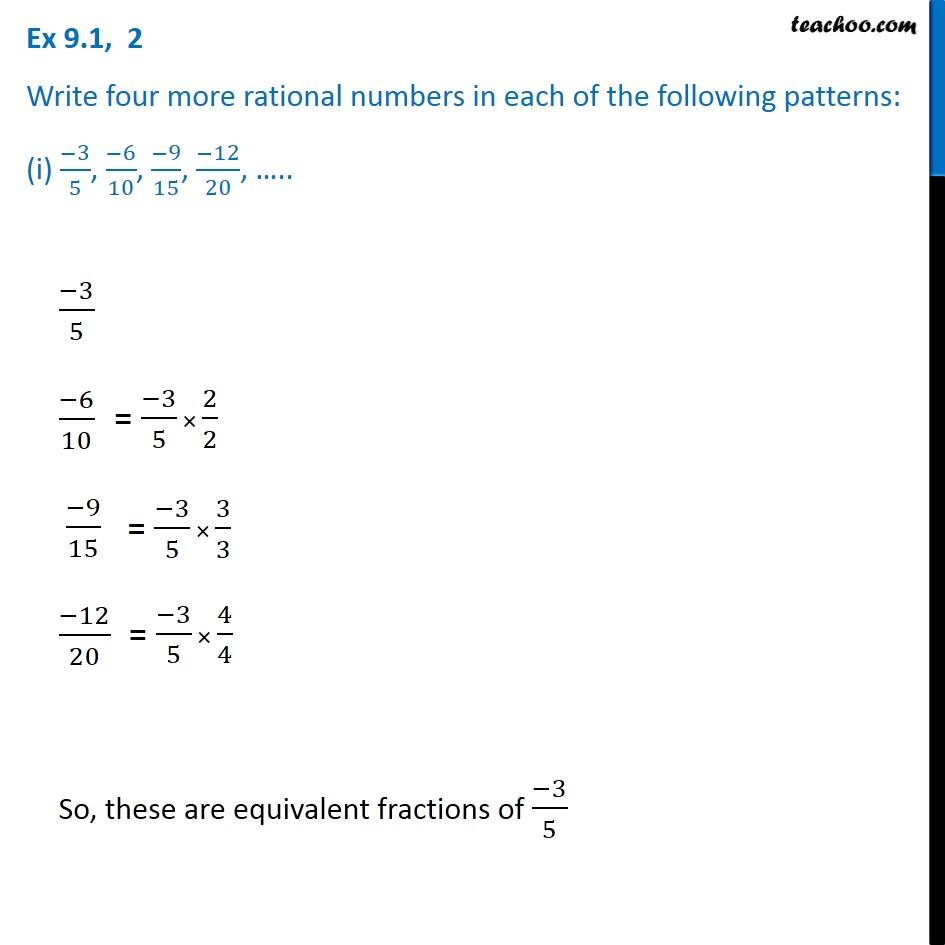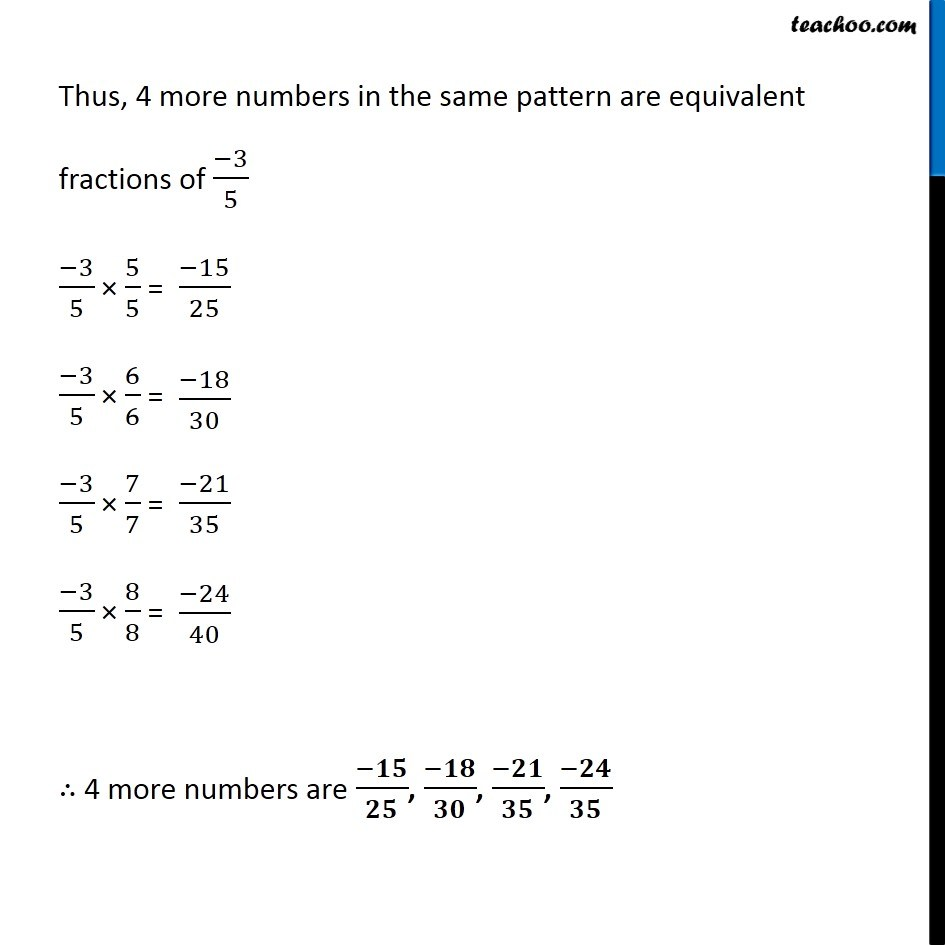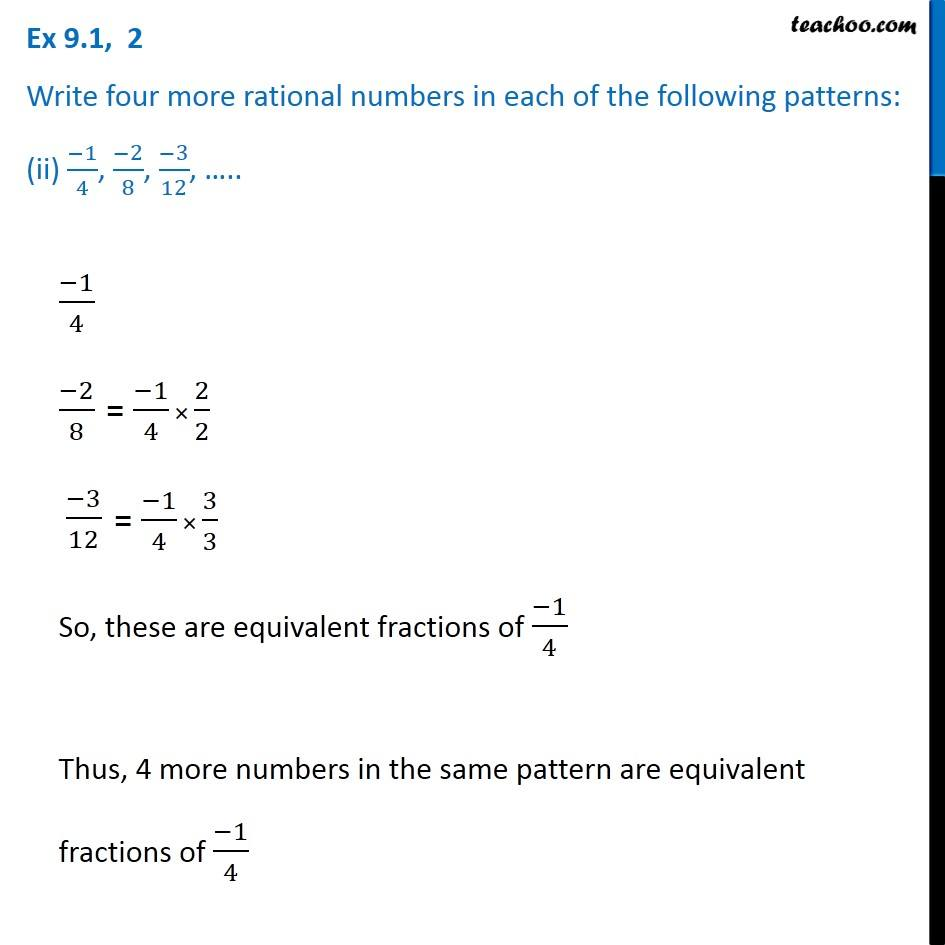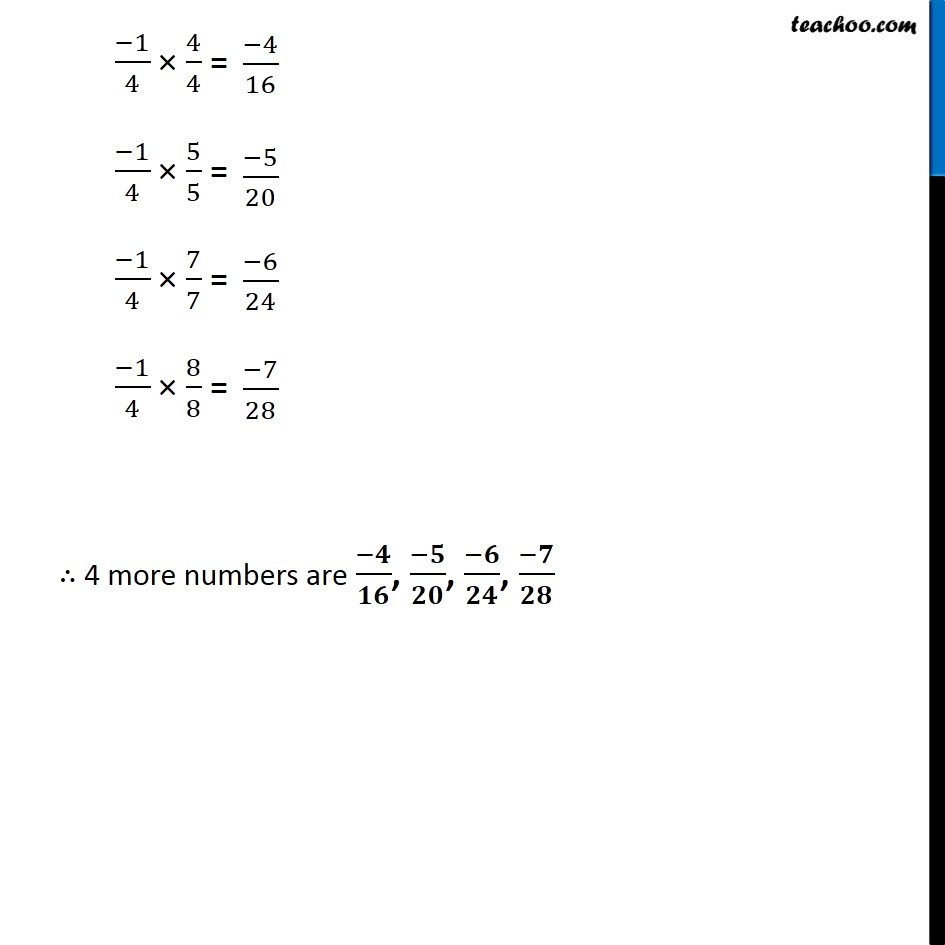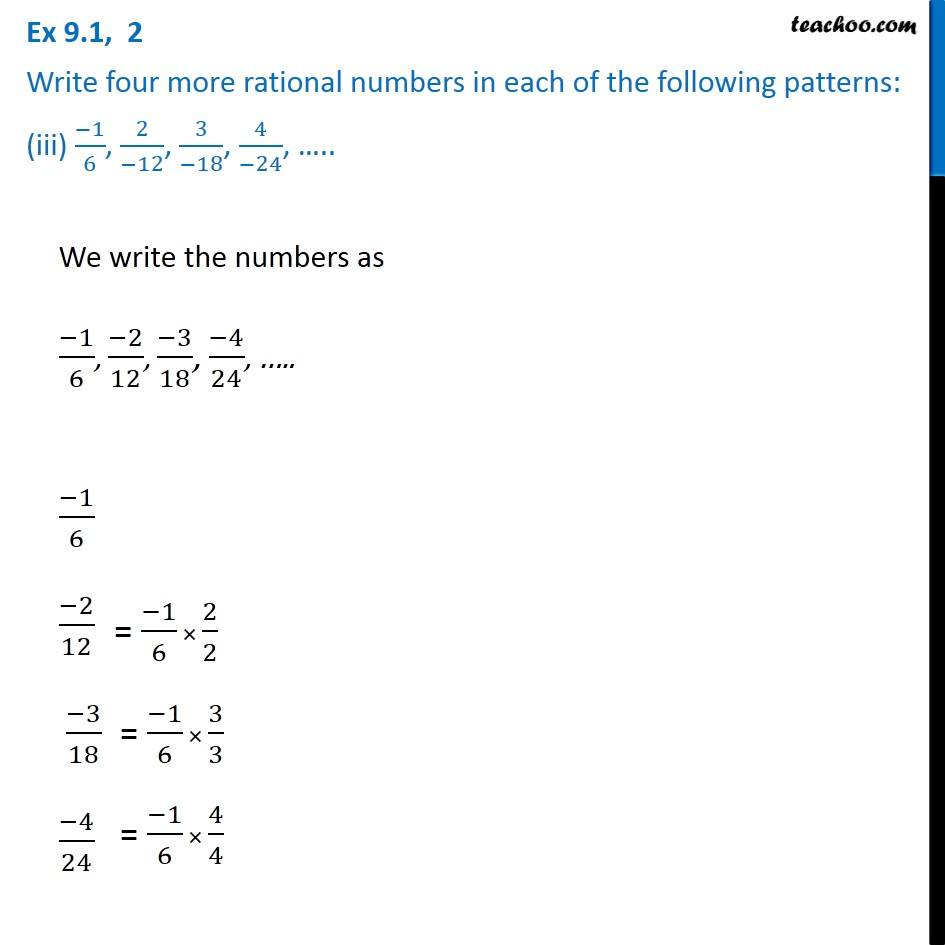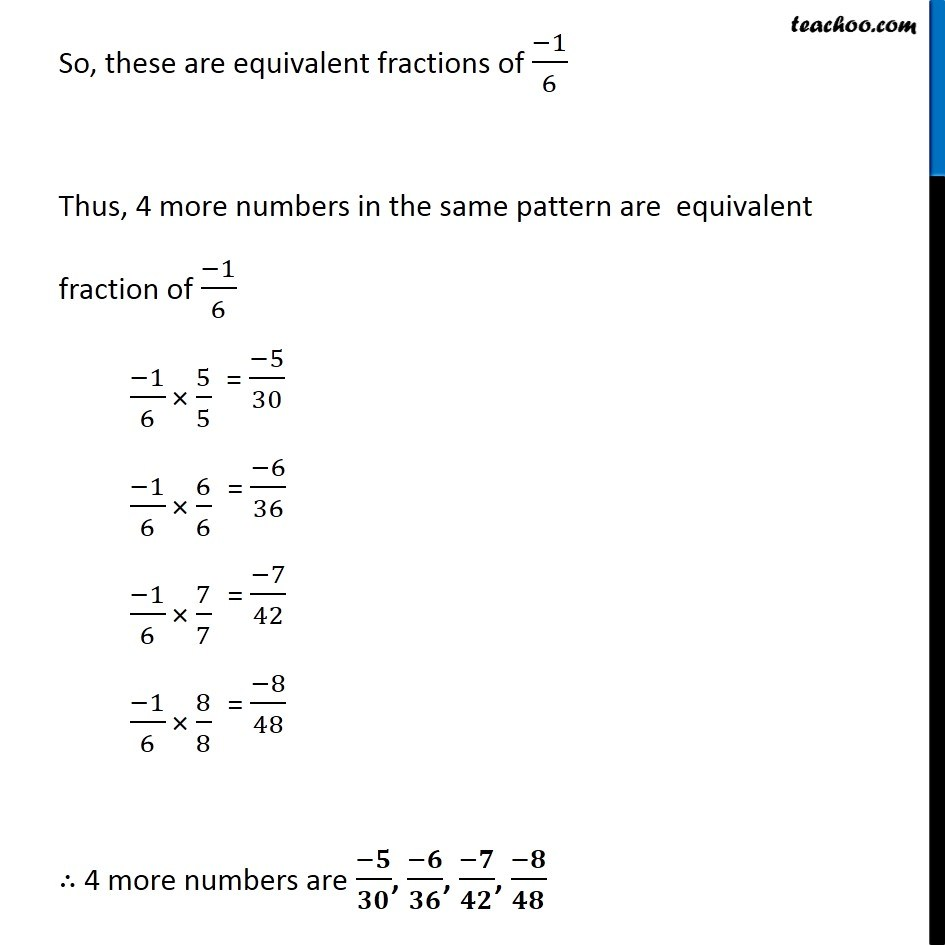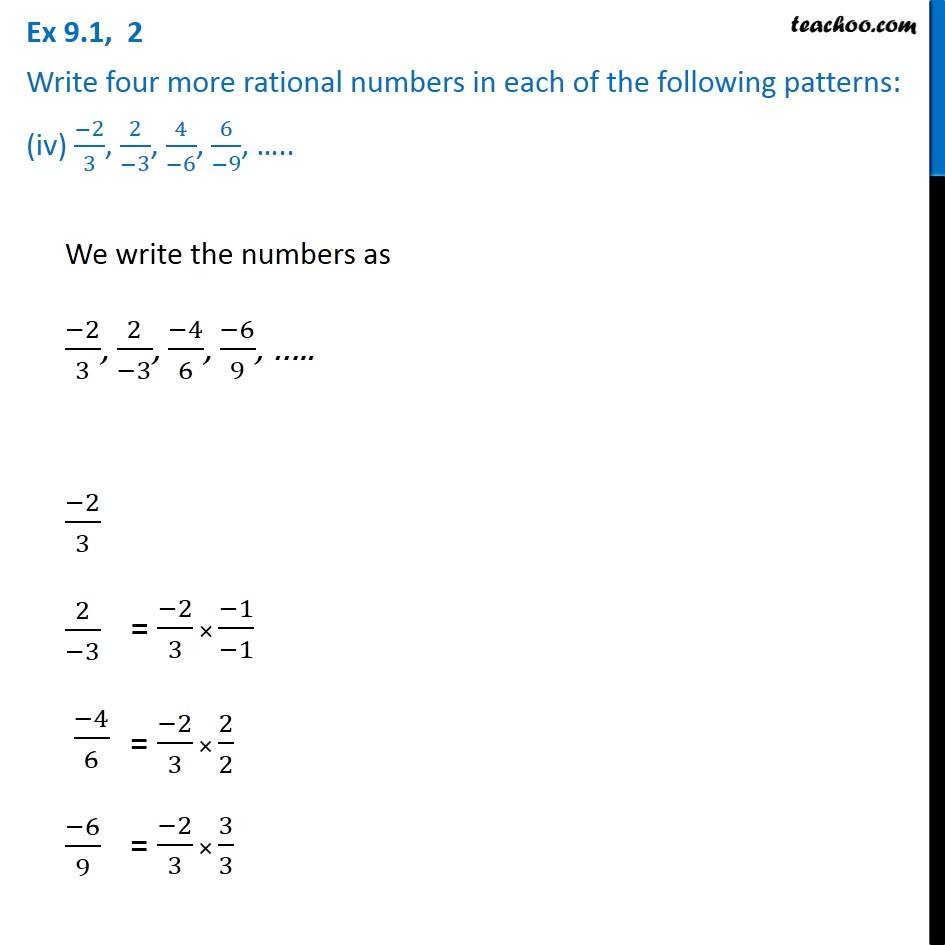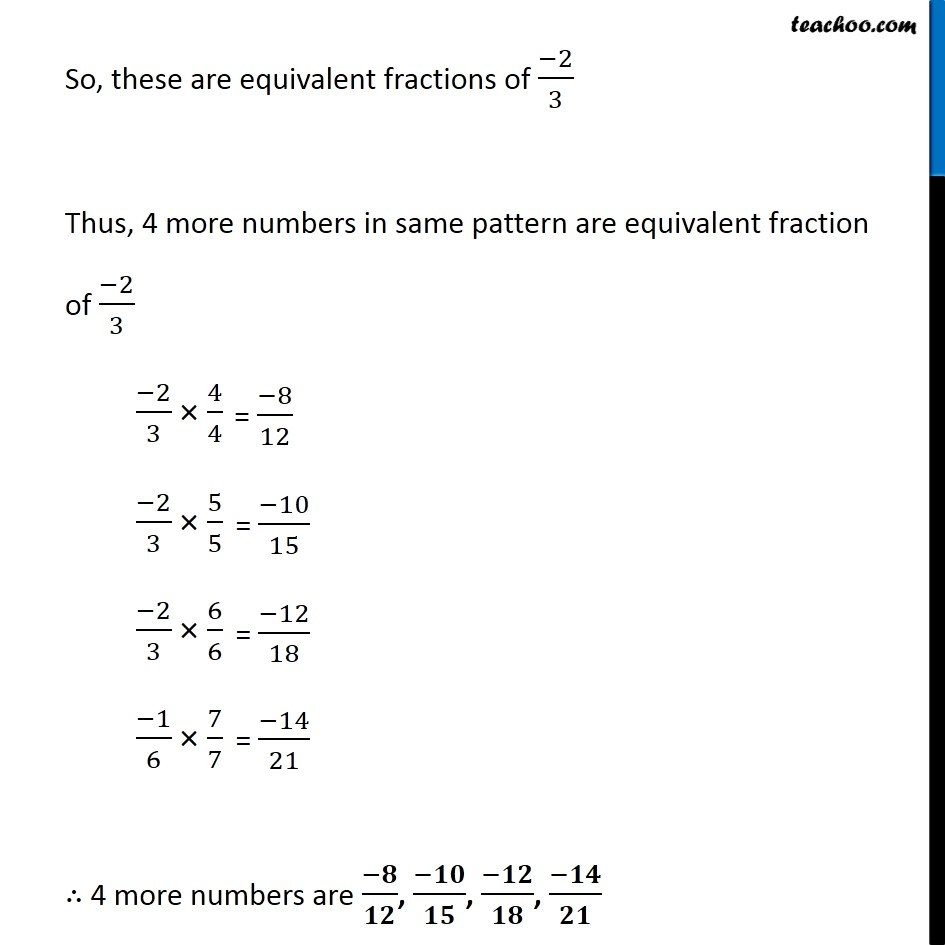1. Chapter 9 Class 7 Rational Numbers
2. Serial order wise
3. Ex 9.1

Transcript

Ex 9.1, 2 Write four more rational numbers in each of the following patterns: (i) (−3)/5, (−6)/10, (−9)/15, (−12)/20, ….. (−3)/5= (−3)/5 × 2/2 (−6)/10 = (−3)/5 × 3/3 (−9)/15 (−12)/20 = (−3)/5 × 4/4 So, these are equivalent fractions of (−3)/5 Thus, 4 more numbers in the same pattern are equivalent fractions of (−3)/5 (−3)/5 × 5/5 = (−3)/5 × 6/6 = (−3)/5 × 7/7 = (−3)/5 × 8/8 = ∴ 4 more numbers are (−𝟏𝟓)/𝟐𝟓, (−𝟏𝟖)/𝟑𝟎, (−𝟐𝟏)/𝟑𝟓, (−𝟐𝟒)/𝟑𝟓 Ex 9.1, 2 Write four more rational numbers in each of the following patterns: (ii) (−1)/4, (−2)/8, (−3)/12, ….. (−1)/4 (−2)/8 (−3)/12 So, these are equivalent fractions of (−1)/4 Thus, 4 more numbers in the same pattern are equivalent fractions of (−1)/4 (−1)/4 × 4/4 = (−1)/4 × 5/5 = (−1)/4 × 7/7 = (−1)/4 × 8/8 = ∴ 4 more numbers are (−𝟒)/𝟏𝟔, (−𝟓)/𝟐𝟎, (−𝟔)/𝟐𝟒, (−𝟕)/𝟐𝟖 Ex 9.1, 2 Write four more rational numbers in each of the following patterns: (iii) (−1)/6, 2/(−12), 3/(−18), 4/(−24), ….. We write the numbers as (−1)/6, (−2)/12, (−3)/18, (−4)/24, ….. (−1)/6 (−2)/12 (−3)/18 (−4)/24 So, these are equivalent fractions of (−1)/6 Thus, 4 more numbers in the same pattern are equivalent fraction of (−1)/6 (−1)/6 × 5/5 (−1)/6 × 6/6 (−1)/6 × 7/7 (−1)/6 × 8/8 ∴ 4 more numbers are (−𝟓)/𝟑𝟎, (−𝟔)/𝟑𝟔, (−𝟕)/𝟒𝟐, (−𝟖)/𝟒𝟖 Ex 9.1, 2 Write four more rational numbers in each of the following patterns: (iv) (−2)/3, 2/(−3), 4/(−6), 6/(−9), ….. We write the numbers as (−2)/3, 2/(−3), (−4)/6, (−6)/9, ….. (−2)/3 2/(−3) (−4)/6 (−6)/9 So, these are equivalent fractions of (−2)/3 Thus, 4 more numbers in same pattern are equivalent fraction of (−2)/3 (−2)/3 × 4/4 (−2)/3 × 5/5 (−2)/3 × 6/6 (−1)/6 × 7/7 ∴ 4 more numbers are (−𝟖)/𝟏𝟐, (−𝟏𝟎)/𝟏𝟓, (−𝟏𝟐)/𝟏𝟖, (−𝟏𝟒)/𝟐𝟏

Ex 9.1